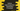# Logical operators in Python : Explanation with example## What are logical operators in python :

The logical operation is mainly done with conditional statements. These are mainly used with two logical operands if the value of logical operands is either True or False. The result of the logical operator is used for the final decision making. Three different types of logical operators are available in python:

1. OR or Logical OR
2. AND or Logical AND
3. NOT or Logical NOT

### Logical OR :

The output of logical OR will be False only if both operands are False. If either of them has a True value, it will result True. The syntax ‘or’  is used for logical OR operation. Following are the input and result of different OR operations : As you can see that the final result is ‘False’ only if both operands are False. Else, it is True always.

### Logical AND :

The output of logical AND will be True only if both operands are True. If anyone of them is False, the result will be False. Syntax ‘and’ is used for logical AND operation. Input and results for different AND operations are as follow :

The final output is True _only if both operands are _True. Else, it is False.

### Logical NOT :

logical NOT is simple. It will just reverse the value. If the input is True, it will return False and if the input is False, it will return True. So, if the value is True, NOT will change it to False and if it is False, NOT will change it to True.

### Example :

Let’s try to learn how logical operator works with a simple python example :

``````#1
num = int(input("Enter a number : "))

#2
if(num > 9 and num < 100):
print("It is a two digit number")
else:
print("It is not a two digit number")

#3
if(num%2 == 0 or num%3 == 0):
print("It is divisible by either 2 or 3")
else:
print("It is not divisible by 2 and 3")

#4
if(not num%5 == 0):
print("It is not divisible by 5")
else:
print("It is divisible by 5")``````